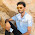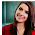# Computer System Architecture Set 7

### Questions 61 to 70

61.
Convert decimal value (888)10 to base-5.
 (a) (444)5 (b) (12023)5 (c) (131313)5 (d) (12021)5 (e) (31320)5.
62.
Consider the following logic function f(ABC).
f(A,B,C) =  ( A + B + C’) . (A + B’+ C’)
Which of the following would be the result if the above logic function is to be simplified using k-maps?
 (a) C’ + A (b) C + C’A’ (c) C.A’ (d) C’.A (e) C’.A’ + B.
63.
What is the equivalent in hexadecimal for the decimal number 973?
 (a) 4BC (b) CB4 (c) 6D (d) 3CD (e) 4CD.
64.
What is the word size of a 8086 processor?
 (a) 8 bits (b) 16 bits (c) 32 bits (d) 64 bits (e) 128 bits.
65.
What kind of Information is stored inside the computer?
 (a) Binary form (b) ASCII code form (c) Decimal form (d) Alpha numeric (e) Numeric form.
66.
What is a parity bit?
 (a) It is used to indicate uppercase letters (b) It is used to detect errors (c) It is the first bit in a byte (d) It is the last bit in a byte (e) It is used to indicate lowercase letters.
67.
What is the minimum number of bits required to store the Hexadecimal number FF?
 (a) 2 (b) 4 (c) 8 (d) 16 (e) 32
68.
What is the purpose of the floating point unit(FPU)?
 (a) Makes integer arithmetic faster (b) Makes pipelining efficient (c) Increases RAM capacity (d) Makes some arithmetic calculations faster (e) Decreases RAM capacity.
69.
Which of the following is not a type of processor?
 (a) PowerPC 601 (b) Motorola 8086 (c) Motorola 68000 (d) Intel Pentium (e) Z80.
70.
Cycle stealing is/are used in which concept?
 (a) Programmed I/O (b) DMA (c) Interrupts (d) Memory mapped I/O (e) All of the above.

#### Answers

 61 Answer :   (b) Reason  :  Divide the given number 888 by 5 and write all the remainder terms from the bottom to up and finally we will get (12023) to the base 5 62 Answer :   (a) Reason  :  C’ + A 63 Answer :   (d) Reason  :  3CD 64 Answer :   (b) Reason  :  8086 is a 16 bit processor. 65 Answer :   (a) Reason  :  The data inside a computer is represented in Binary form i.e (0’s and 1’s). 66 Answer :   (b) Reason  :  A parity bit is used to detect errors. 67 Answer :   (b) Reason  :  The minimum number of bits required to store a Hexadecimal number FF is 4. 68 Answer :   (d) Reason  :  Makes some arithmetic calculations faster. 69 Answer :   (b) Reason  :  Motorola 8086 is not a processor. 70 Answer :   (b) Reason  :  Cycle Stealing concept is used in DMA .

29  30  31  32  33  34  35  36  37  38  39  Next >>

#### 4 comments :

1.Q:67 8 bits are required to store FF not 4.
Q63: equivalent hexadecimal of 973 is 6D not 3CD

2.more question repeating

3.It has been simply incredibly generous with you to provide openly what exactly many individuals would’ve marketed for an eBook to end up making some cash for their end, primarily given that you could have tried it in the event you wanted.

AWS Training in Bangalore

4.Hey, would you mind if I share your blog with my twitter group? There’s a lot of folks that I think would enjoy your content. Please let me know. Thank you.
AWS Training in Chennai
Data Science Training in Chennai
Python Training in Chennai
RPA Training in Chennai
Digital Marketing Training in Chennai